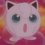An image of small size can be obtained on a screen placed at 2.0 m from object. It can be done by using:

(A) concave mirror of focal length 0.5 m
(B) concave mirror of focal length greater than 0.5 m
(C) concave mirror of focal length less than 0.5 m
(D) concave lens of focal length less than 0.5 m.

Can you provide a good solution for this? Thank youNote by Sahba Hasan
6 years ago

This discussion board is a place to discuss our Daily Challenges and the math and science related to those challenges. Explanations are more than just a solution — they should explain the steps and thinking strategies that you used to obtain the solution. Comments should further the discussion of math and science.

When posting on Brilliant:

• Use the emojis to react to an explanation, whether you're congratulating a job well done , or just really confused .
• Ask specific questions about the challenge or the steps in somebody's explanation. Well-posed questions can add a lot to the discussion, but posting "I don't understand!" doesn't help anyone.
• Try to contribute something new to the discussion, whether it is an extension, generalization or other idea related to the challenge.

MarkdownAppears as
*italics* or _italics_ italics
**bold** or __bold__ bold
- bulleted- list
• bulleted
• list
1. numbered2. list
1. numbered
2. list
Note: you must add a full line of space before and after lists for them to show up correctly
paragraph 1paragraph 2

paragraph 1

paragraph 2

[example link](https://brilliant.org)example link
> This is a quote
This is a quote
    # I indented these lines
# 4 spaces, and now they show
# up as a code block.

print "hello world"
# I indented these lines
# 4 spaces, and now they show
# up as a code block.

print "hello world"
MathAppears as
Remember to wrap math in $$ ... $$ or $ ... $ to ensure proper formatting.
2 \times 3 $2 \times 3$
2^{34} $2^{34}$
a_{i-1} $a_{i-1}$
\frac{2}{3} $\frac{2}{3}$
\sqrt{2} $\sqrt{2}$
\sum_{i=1}^3 $\sum_{i=1}^3$
\sin \theta $\sin \theta$
\boxed{123} $\boxed{123}$

Sort by:

What have you tried? What do you know?

Staff - 6 years ago

I know that option D is not true because a concave lens won't produce an image which can be obtained on a screen, i.e it won't produce a real image. The things which i can't figure out are the three options left. In the question the object distance is not given. 2.0 m is mere distance of screen from the object, i.e. image from the object.

How can i figure out the focal length of the mirror?

- 6 years ago

- 6 years ago

B will be the option as in that case, the object will be placed between centre of curvature and focal length. Since the object is placed at 2 m away from the mirror, and it has to be in between Curvature and focus. The centre of curvature therefore should be greater than 2 m which makes focal length automatically greater than 0.5 m.

- 6 years ago

You got it wrong @Abhay Tiwari ...The object distance is not given, the distance of the image from the object is given.

- 6 years ago

Still the answer remains B :p

- 6 years ago

I can't understand how...@Abhay Tiwari ...

- 6 years ago